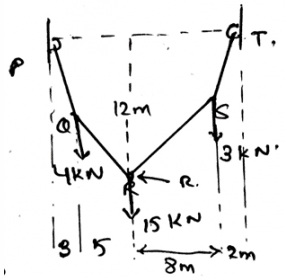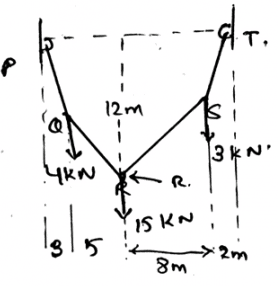Courses

# Test: Cables

## 15 Questions MCQ Test Engineering Mechanics | Test: Cables

Description
This mock test of Test: Cables for Mechanical Engineering helps you for every Mechanical Engineering entrance exam. This contains 15 Multiple Choice Questions for Mechanical Engineering Test: Cables (mcq) to study with solutions a complete question bank. The solved questions answers in this Test: Cables quiz give you a good mix of easy questions and tough questions. Mechanical Engineering students definitely take this Test: Cables exercise for a better result in the exam. You can find other Test: Cables extra questions, long questions & short questions for Mechanical Engineering on EduRev as well by searching above.
QUESTION: 1

### Flexible cable with chains combine ___________ with lightness and often are used in structures for support.

Solution:

The chains with the cables are used to support the structures. They ae there for the transfer of the loading from one point to another point. This means that the cables not only provides strength to the structure but also sufficiently transfers the loads that are being added to it.

QUESTION: 2

### In the support system of the bridges and trolley wheel ____________ form the main loading carrying element in the structure.

Solution:

The cable is a support system which is used to transfer the loadings in the different structures. The main motto is to make the structure stable. This is probably done with the help of making the distribution of the load which is being given to the structures equally by the help of the cables.

QUESTION: 3

### The force on the cables is not neglected in the calculations of the load distribution.

Solution:

The cable is a support system which is used to transfer the loadings in the different structures. The main motto is to make the structure stable. In calculation the self-weight of the cable is neglected and the load is calculated. The forces are acting in the vertically downward direction.

QUESTION: 4

The cable weight become significant in the calculations of the loadings when the cables are used in the transmission lines and guys for radio antennas.

Solution:

The cable is a support system which is used to transfer the loadings in the different structures. The main motto is to make the structure stable. But in the calculations of the loadings when the cables are used in the transmission lines and guys for antennas the self-weight of the cable is not neglected and the load is calculated.

QUESTION: 5

The assumptions for the calculations are done for the cables. In that one of the assumption is that the cable is ___________

Solution:

The assumptions are done so as to make the calculations easy. Though the assumptions make the small errors to not count over the big dat. But still if the assumptions are made then the calculations are easy. Thus the assumption taken as the cable is perfectly flexible.

QUESTION: 6

The assumptions for the calculations are done for the cables. In that one of the assumption is that the cable is flexible and the other is that the cable is ___________

Solution:

The assumptions are done so as to make the calculations easy. Though the assumptions make the small errors to not count over the big dat. But still if the assumptions are made then the calculations are easy. Thus the assumption taken as the cable is inextensible.

QUESTION: 7

Determine the force in the section QR as shown in the figure.Solution:

The various points in the cables are facing constant forces. The forces are tensile forces. This is because as the cables are subjected to loadings the loads tends to stretch the cables in the direction parallel to its round area region. Thus the points face tensile forces which are parallel to the cable length.

QUESTION: 8

Due to which property the cable, it offers no resistance to bending?

Solution:

Due to the flexibility property the cable, it offers no resistance to bending. As the bending is seen in the beams and all the solid structures. Thus the bending moment produced in the cables are not affecting the cables much. Thus no affect by bending, i.e. no resistance to bending.

QUESTION: 9

The tensile force acting on the cable is in which direction w.r.t the cable?

Solution:

Due to the flexibility property the cable, it offers no resistance to bending. As the bending is seen in the beams and all the solid structures. Thus the bending moment produced in the cables are not affecting the cables much. So the tensile force which is being produced is acting in the tangential direction to the points of the cable along its lengths.

QUESTION: 10

Being inextensible the cable has ___________ length.

Solution:

The assumption that the cables are inextensible the main fact that comes out is that the cable length is constant throughout. Thus in the calculations also the fact that the length is constant makes a huge time saving tool. Thus the length of the cables are to be assumed constant if the assumption is taken as they are inextensible.

QUESTION: 11

The loading in the cable doesn’t changes the ___________ of the cables.

Solution:

The loading in the cables doesn’t affect the geometry of the cables. This is because of the assumptions which we have taken. The first one that the cables are perfectly elastic. And the second one that the cables are inextensible.

QUESTION: 12

Cable takes a shape of a ____________ when is subjected to loadings.

Solution:

The cable takes the shape of straight line when subjected to the loadings. As the loadings are straight, acting vertically downwards, they stretch the cables and then make them come in the shape of the straight line. Thus the loadings make the cables come in the straight line.

QUESTION: 13

The various points in the cable is facing a ____________ tensile force.

Solution:

The various points in the cables are facing constant forces. The forces are tensile forces. This is because as the cables are subjected to loadings the loads tends to stretch the cables in the direction parallel to its round area region. Thus the points face tensile forces which are parallel to the cable length.

QUESTION: 14

If the unknown variables in the calculations are more than the known quantities, then the number of equations required to solve all the unknown variables are?

Solution:

Whatever be the calculation involved, the unknown variables will be ultimately come out from the equations. The proper use of the known quantities and the multiuse of various calculation techniques will ultimately give up the results. Thus whether it may be the case of the cables or the beams the equations if applied properly will result in the determination of the unknowns.

QUESTION: 15

Determine the force in the section PQ as shown in the figure.Solution:

The various points in the cables are facing constant forces. The forces are tensile forces. This is because as the cables are subjected to loadings the loads tends to stretch the cables in the direction parallel to its round area region. Thus the points face tensile forces which are parallel to the cable length.